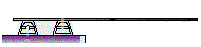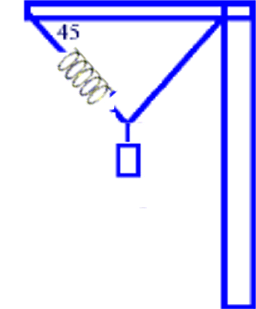### Tips for solving Torque and Static equilibrium problems

1. Analyze the Problem (read it several times -- identify the Given & the Required)
2. Draw the Free Body Diagram (FBD)
3. Include all forces
4. Include Fg at the center of mass -- when conditions are symmetrical
5. Assign all Torques
6. Assign the correct sign (+) or (-) to Force Vectors and Torque Vectors
7. (-) Force x (+) distance = (-) Torque
8. (+) Force x (+) distance = (+) Torque
9. (+) Force x (-) distance = (-) Torque
10. Apply the three conditions for Static Equilibrium
11. ΣFx = 0;  ΣFy = 0; Σt = 0
12. Minimize the number of unknown variables by placing the reference point at one of the Forces

#### Review Problems: Rotational Motion, Torque and Static equilibrium

1. In the design of a roller coaster the maximum speed necessary to keep the passengers from falling out of the cart when traveling upside down is  9.0 m/s.  Calculate the centripetal acceleration and the diameter of the loop.

2. At what height above the earth’s surface must a satellite be placed if it is to remain over the same geographical point on the equator of the earth?  G = 6.67 X 10 -11 Nm2/Kg2    Mass of Earth = 5.98 X 1024 Kg   Radius of Earth = 6.37 X 106 m
3. A  12 m aluminum ladder of mass 10.0 Kg leans against a smooth wall so that the force exerted by the wall is perpendicular to the wall.  The ladder makes a 100 with the vertical wall; and the ground is rough.  Calculate :
a)  The components of the force exerted by the ground on the ladder.
b)  The coefficient of friction at the base of the ladder if the ladder is not to slip when a 65 kg person stands three-quarters of the way up the ladder.
4. The forces on the supports at the left hand of the cantilever are equal.  The mass of the cantilever is 40 Kg.  What is the maximum mass standing at the tip of the cantilever than can be supported without upsetting the equilibrium of the system?5. By what amount will the spring stretch when a 20 Kg mass is suspended from it.
The spring constant is 10 000 N/m -- see diagram below.6. At what minimum speed should a bucket full of water be twirled (rotated) in a vertical circle to prevent any water in it from escaping from it?